Refer to our Texas Go Math Grade 2 Answer Key Pdf to score good marks in the exams. Test yourself by practicing the problems from Texas Go Math Grade 2 Module 14 Assessment Answer Key.

Concepts and Skills

Use a ruler to draw the shape. TEKS 2.8.A

Question 1.
a shape with 5 sides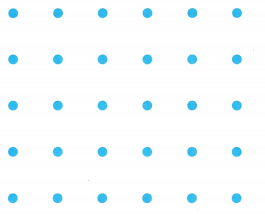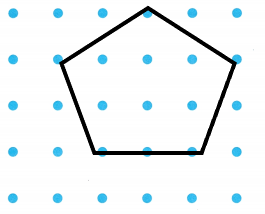Explanation:
I drew a shape that has 5 sides.It is a pentagon.

Question 2.
a shape with 4 verticesExplanation:
I drew a rectangle as it has 4 vertices.

Question 3.
Combine the pattern blocks. Trace the blocks to show how you made the new shape. TEKS 2.8.D
Make a new shape that has 6 vertices.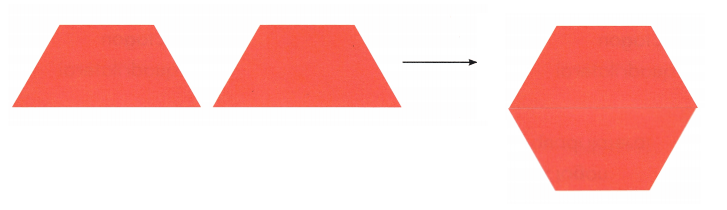Explanation:
I combined the pattern blocks and made a hexagon that has 6 sides.

Question 4.
Circle the shapes with more than 4 vertices. TEKS 2.8.CExplanation:
I drew a circle around the shapes that has more than 4 vertices.

Texas Test Prep

Fill in the bubble for the correct answer choice.

Question 5.
Dev drew this pentagon. How many vertices does a pentagon have? TEKS 2.8.C(A) 5 vertices
(B) 4 vertices
(C) 6 vertices
(A) 5 vertices

Explanation:
Dev drew this pentagon.A pentagon has 5 vertices.

Question 6.
Ella drew this polygon. How many sides does this polygon have? TEKS 2.8.C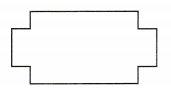(A) 8 sides
(B) 12 sides
(C) 13 sides
(B) 12 sides

Explanation:
Ella drew this polygon.This has 12 sides.

Question 7.
Paulo drew this shape. What is the name of the shape? TEK 2.8.C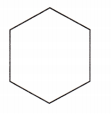(A) hexagon
(B) octagon
(A) Hexagon

Explanation:
Paulo drew this shape.It is a hexagon it has 6 sides.

Question 8.
Barbara folded the shape along the line. What new shapes did she make? TEKS 2.8.E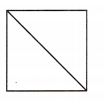(A) 2 squares
(B) 1 square, 1 triangle
(C) 2 triangles